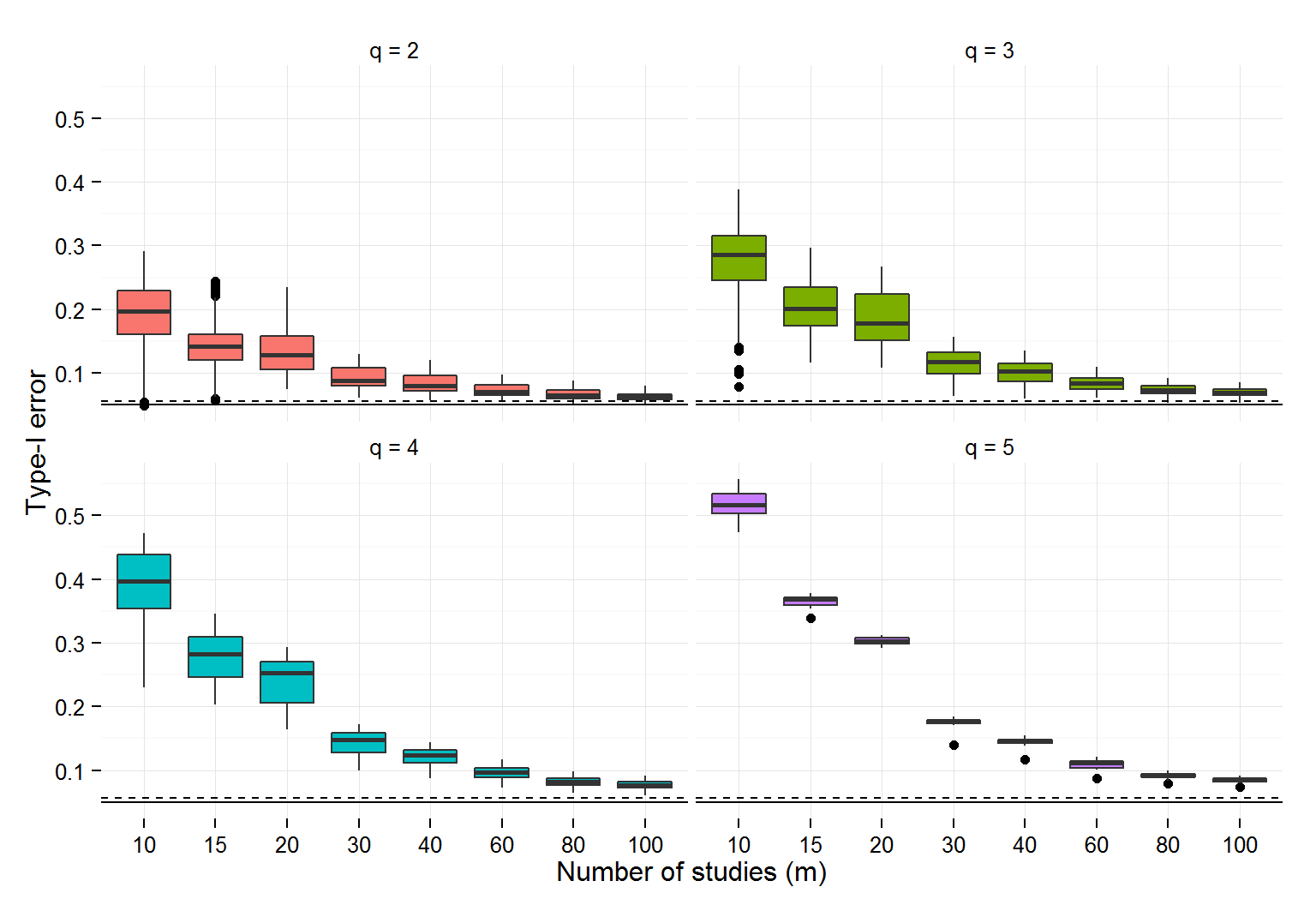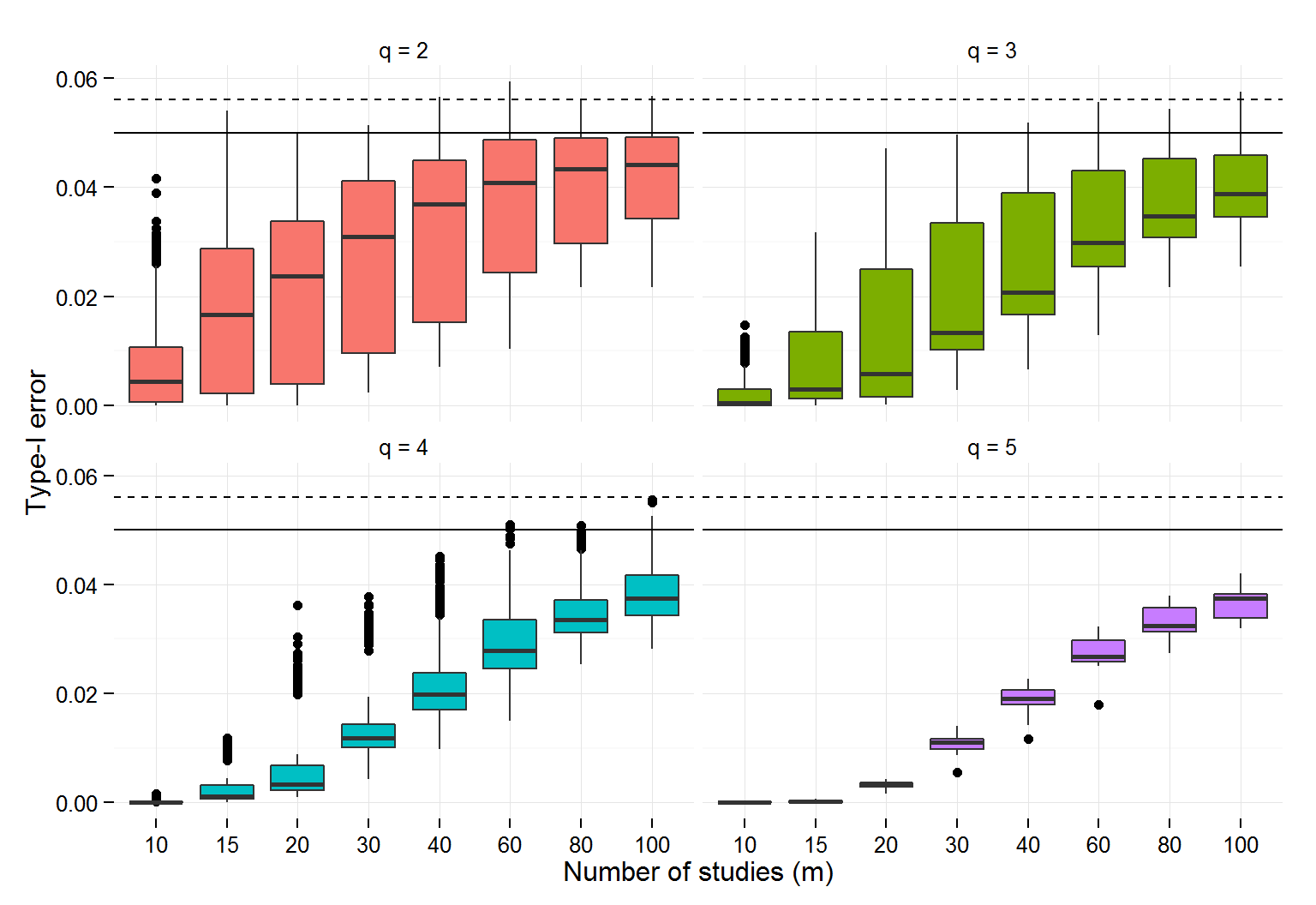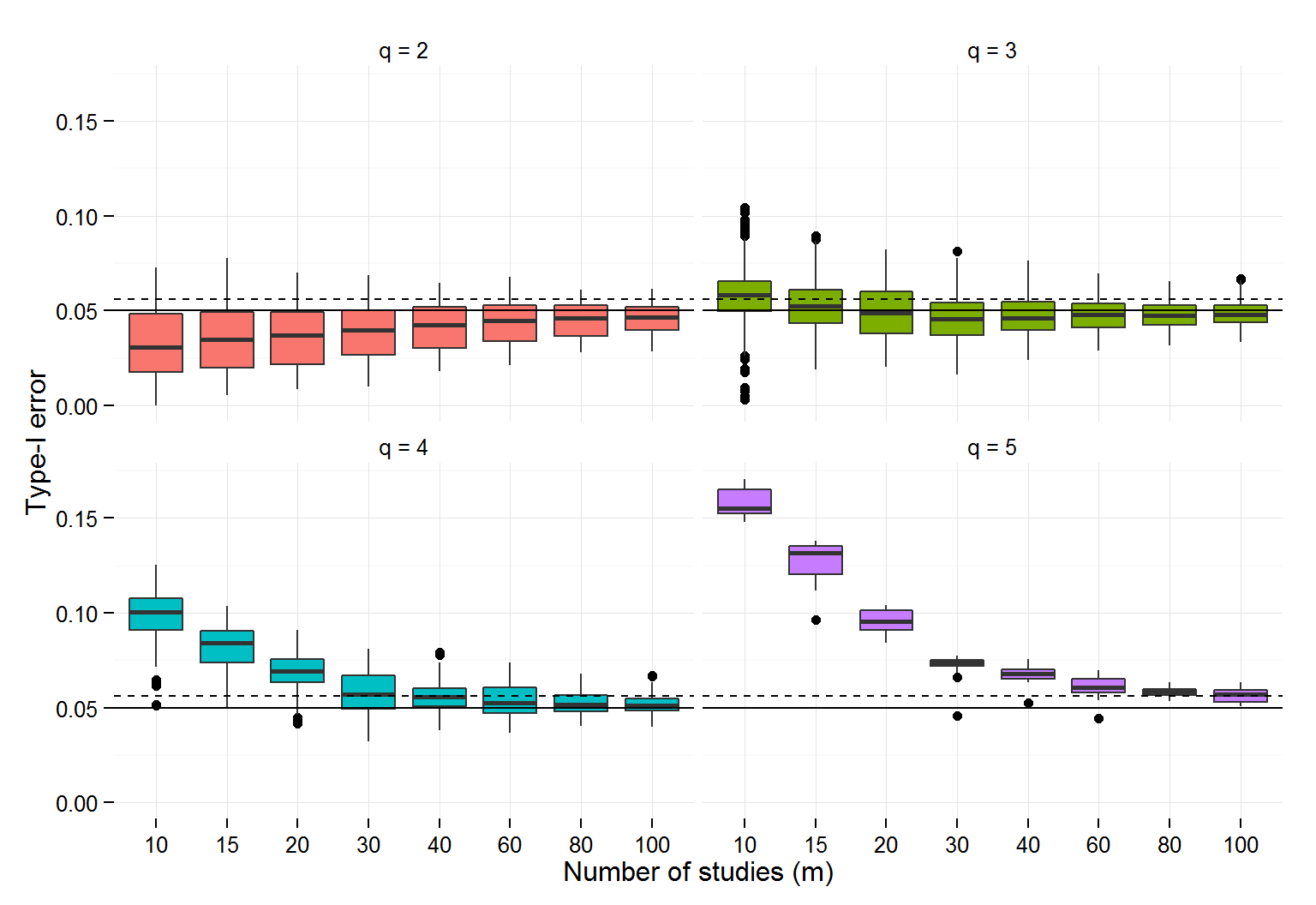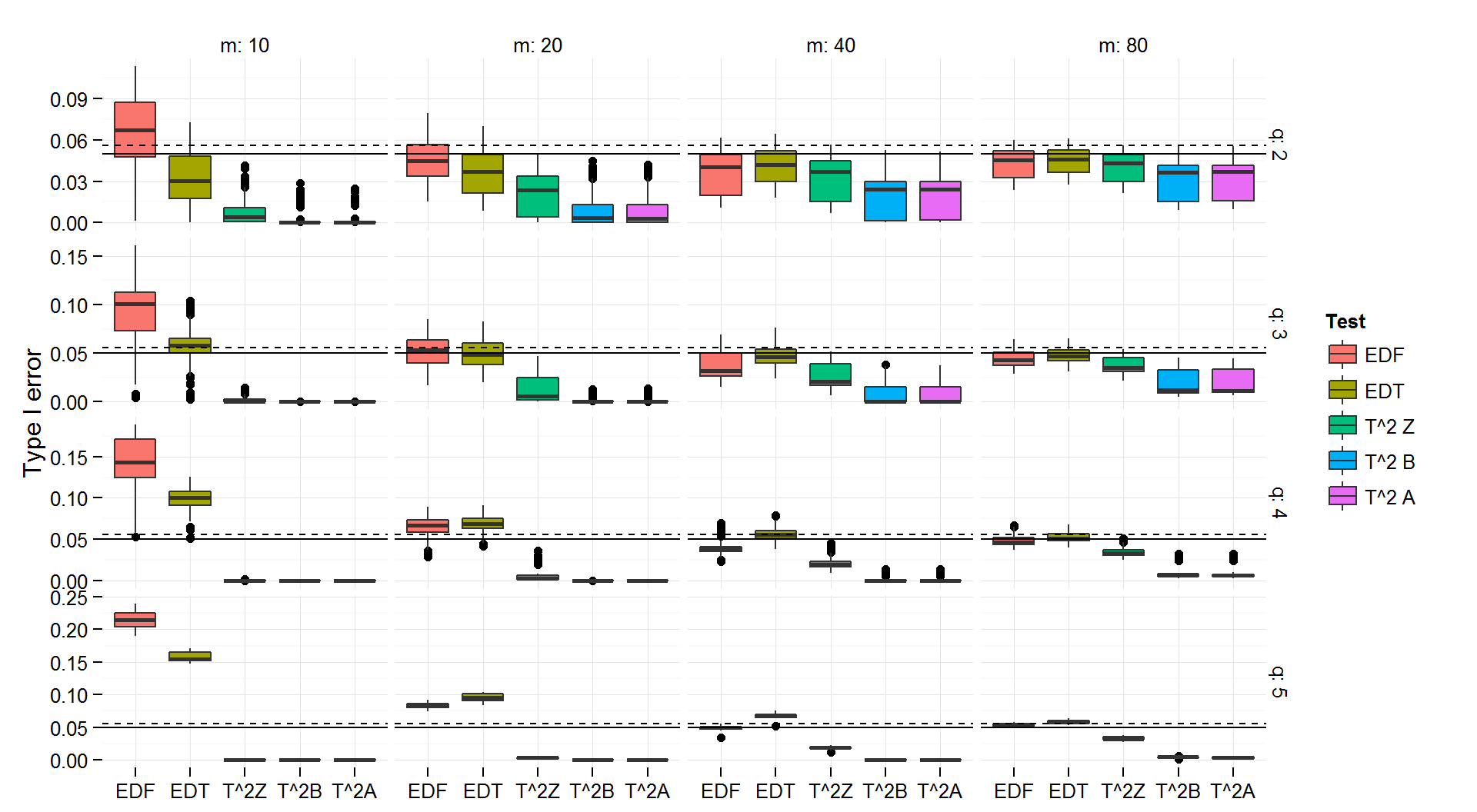March 6, 2015

## Meta-analysis with dependent effect sizes

• Meta-analysis is a set of tools for synthesizing results from many different sources
• Studies often report multiple effect sizes
• Two methods for handling dependent effects:
• Univariate meta-analysis, where each study contributes a single effect size, or
• Multivariate meta-analysis, where the covariance structure of the multiple effect sizes is known.
• Neither approach is ideal

## Robust variance estimation

Robust variance estimation (RVE; Hedges, Tipton, & Johnson, 2010) produces asymptotically valid standard errors and hypothesis tests, even if the error structure is mis-specified.

• Accurate estimates of correlations between effect sizes are not needed.
• Meta-regressions estimated by weighted least squares (just like model-based multivariate meta-regression).
• Weights can be based on effect size variances and rough imputations of correlations.
• But exact inverse-variance weights are not needed.
• Variances of meta-regression coefficients estimated using a "sandwich" formula (Liang & Zeger, 1986).

## Hypothesis testing

In large samples, we can use RVE to construct hypothesis tests.

• For testing a single meta-regression coefficient, the z statistic $$\left(\frac{\text{estimate}}{\text{robust SE}}\right)$$ follows a standard normal distribution if $$m$$ is "big enough."
• Tipton (in press) devised small-sample corrections for t-tests. These corrections involve two parts:
• Adjustments to the robust variance estimator
• Estimated degrees of freedom for the t-distribution (using a Satterthwaite approximation)
• These degrees of freedom differ for each covariate in the model.

## Tests of multiple meta-regression coefficients

• Meta-analysts will often need to test hypotheses involving more than one meta-regression coefficient.
• Test equality of several levels of a moderator
• Test of overall model fit
• For simulatenously testing several meta-regression coefficients, one can use a Wald statistic $Q = \left(\text{estimates}\right)' \left(\text{RVE matrix}'\right)^{-1} \left(\text{estimates}\right)$
• In large samples, we would expect $$Q$$ to follow a chi-squared distribution with $$q$$ degrees of freedom.
• Simulation results suggest that this test can have severely inflated Type I error.

## Small sample F-tests

We follow a two-part strategy for constructing small-sample tests:

• Adjustments to the robust variance estimator
• based on McCaffrey, Bell, & Botts' (2001) "bias-reduced linearization" approach.
• Estimated degrees of freedom for the F-distribution
• but how to estimate these degrees of freedom?
• Drawing on extant literature, we investigated a wide variety of possible corrections.
• Fai-Cornelius (1996): mixed models
• Cai-Hayes (2008): heteroskedasticity robust standard errors
• Zhang (2012, 2013): heteroskedastic ANOVA/MANOVA
• Pan-Wall (2002): generalized estimating equations

## The Winner: $$T^2_Z$$

• The paper provides results for five different corrections. Here, however, we'll focus on only the one that works best.
• The $$T^2_Z$$ approach $c \times Q/q \sim F(q, df)$ where $$df$$ is estimated by matching the mean and total variance of the RVE matrix.
• The $$T^2_Z$$ is best in two regards
• It is (almost) always level-alpha
• It is more powerful than any of the other level-alpha estimators (i.e., always has error rates closer to nominal)

## Simulated type-I error rate of $$\chi^2$$ test## Simulation results: $$T^2_Z$$## Example: Wilson et al. (2011)

• Wilson, Lipsey, Tanner-Smith, Huang, & Steinka-Fry (2011) synthesis of effects of dropout prevention/intervention programs.
• Primary outcomes: school completion, school dropout
• $$m = 152$$ studies, containing 385 effect size estimates
• Meta-regression model including several categorical moderators
Moderator q Chi-sq p-value T-squared Z d.f. p-value
Study design 2 0.23 0.796 0.22 43 0.800
Outcome measure 3 0.91 0.436 0.84 22 0.488
Evaluator independence 3 3.11 0.029 2.78 17 0.073
Implementation quality 2 14.15 <0.001 13.78 37 <0.001
Program format 3 3.85 0.011 3.65 38 0.021

## Conclusions and future work

• Like Tipton (in press) found with the small-sample t-test…
• The performance of the large-sample test depends on features of the covariates, not just sample size.
• Consequently, it is hard to know a priori what constitutes a "big enough" sample.
• We therefore recommend that small-sample corrections should always be used in practice.
• We provide prototype software in R (upon request), and are working on implementing it fully into the robumeta R package and Stata macro and the metafor R package (Viechtbauer, 2010).

## Simulation results: EDT test## Comparison of small-sample corrections